### ASM to Sega Genesis Platform

Would you like to react to this message? Create an account in a few clicks or log in to continue.
ASM to Sega Genesis Platform

All about assembly programming in the Sega Genesis console.

5 posters

# Homing Attack in Sonic 1 - Step 0 - Theoretical ExplanationOuricoDoido

Mensagens : 46
Data de inscrição : 2011-01-09
Current Project : Sonic Open Source Project
Tutorial to Hivebrain's 2005 disassembly.

If you want to see the final result, download the compiled Rom and the Source Code in this link:
http://goo.gl/hMOUs

What is the Homing Attack?
The Homing Attack is a Sonic's skill, which operates as a directed jump against the enemy, these jumps can be updated, causing the chase effect.

Sonic 1 already have the Homing Attack !!!!!! But the code is programmed for Sonic go in the object's opposite direction.

Where is this code? I never seen ?????In Object 47 - pinball bumper (SYZ), the pinball ball of Spring Yard Zone, see the code, what happens when Sonic hits her.

Code:
`Obj47_Hit:            ; XREF: Obj47_Index      tst.b   \$21(a0)      ; has Sonic touched the   bumper?      beq.w   Obj47_Display   ; if not, branch      clr.b   \$21(a0)      lea   (\$FFFFD000).w,a1      move.w   8(a0),d1      move.w   \$C(a0),d2      sub.w   8(a1),d1      sub.w   \$C(a1),d2      jsr   (CalcAngle).l      jsr   (CalcSine).l      muls.w   #-\$700,d1      asr.l   #8,d1      move.w   d1,\$10(a1)   ; bounce Sonic away      muls.w   #-\$700,d0      asr.l   #8,d0      move.w   d0,\$12(a1)   ; bounce Sonic away`

I want to highlight this part:

Code:
`      lea   (\$FFFFD000).w,a1      move.w   8(a0),d1      move.w   \$C(a0),d2      sub.w   8(a1),d1      sub.w   \$C(a1),d2      jsr   (CalcAngle).l      jsr   (CalcSine).l      muls.w   #-\$700,d1      asr.l   #8,d1      move.w   d1,\$10(a1)   ; bounce Sonic away      muls.w   #-\$700,d0      asr.l   #8,d0      move.w   d0,\$12(a1)   ; bounce Sonic away`
To explain how it works, i divided the code into 3 SECTORS, and explain each one. I created this image to help you understand.1st sector:
Code:
`      lea   (\$FFFFD000).w,a1   ; load the Sonic address to a1      move.w   8(a0),d1   ; move the object x-position to d1      move.w   \$C(a0),d2   ; move the object y-position to d2      sub.w   8(a1),d1   ; sub sonic x-position of object x-position      sub.w   \$C(a1),d2   ; sub sonic y-position of object y-position`
What happens here?
Here the calculation is done to obtain the object's coordinates as if Sonic's coordinates is (x=0, y=0), more precisely the Sonic's distance to the object in the coordinates X (horizontal) and Y (vertical).

But what for?
For calculate the angle, the two points need to stay in the cartesian plane, with X and Y coordinates, and for the CalcAngle, one of the points tends to stay in the coordinate (x=0, y=0).

2nd sector:
Code:
`      jsr   (CalcAngle).l      jsr   (CalcSine).l`
Here the game calculates the angle between the two points, based on values (d1 and d2) that it received in the 1st sector, and calculates the Sine and Cosine based on the angle.

3rd sector:
Code:
`      muls.w   #-\$700,d1      asr.l   #8,d1      move.w   d1,\$10(a1)   ; bounce Sonic away      muls.w   #-\$700,d0      asr.l   #8,d0      move.w   d0,\$12(a1)   ; bounce Sonic away`
For you to understand, you must know the CalcSine's output variables, it uses the d0 for Sine and d1 for Cosine. As the sine and cosine's values are too low, is necessary multiplies them by a higher value.

Why the variable is multiplied by a negative value?
Because, as I said, this is a Homing Attack (directed jump) in the object's opposite direction - is negative for Sonic go in the pinball bumper's opposite direction;

If you want to test, set #\$700 instead of two #-\$700, you'll see that Sonic will go in object's direction (pinball bumper). This test code is equal this:
Code:
`      muls.w   #\$700,d1      asr.l   #8,d1      move.w   d1,\$10(a1)   ; bounce Sonic away      muls.w   #\$700,d0      asr.l   #8,d0      move.w   d0,\$12(a1)   ; bounce Sonic away`
What makes this line?
Code:
`      asr.l   #8,d1`

This line is an Arithmetic Shift Right, how many binary places walk to the right, example:
If this calculation (8 places to the right) is done on the number \$700, this would happen:

Of:
\$700 = %11100000000
To:
\$007 = %00000000111

So, this "asr" can be regarded as a division, the number was divided by \$100 or 2^8.

If you do not want to do this calculation, no need; first, the sine or cosine is multiplied by -\$700, and then, divided by \$100;
could simplify the calculation, dividing -\$700 by \$100, making the code like this:
Code:
`      muls.w   #-\$7,d1      move.w   d1,\$10(a1)   ; bounce Sonic away      muls.w   #-\$7,d0      move.w   d0,\$12(a1)   ; bounce Sonic away`
The base of Homing Attack is this - reverse the effects of pinball bumper's pre-existing programming.

Where to start the Homing Attack?
As you may know, Sonic is a 2D game, and in all 2D games we have the ease of working with the cartesian plane.
The Homing Attack is based on the theory that Sonic needs go toward the enemy.

How is this done?
To know the direction that Sonic should go, we need to know the Sonic's coordinates and the enemy's coordinates, and the Sonic 1 already has a coordinate system, which makes it easy.
The enemy's coordinate is the Sonic's destination point, and the Sonic's coordinate is the Sonic's origin point.

How to know the distance between the object and Sonic, and limit the range of the homing Attack?

How to create a vector between Sonic and the object?
Using the Pythagorean theorem, where the hypotenuse is equal to the adjacent leg squared + opposite leg squared
a^2 = b^2 + c^2

The hypotenuse equals the line between Sonic and the object, the hypotenuse in the first case we use to see if Sonic is near or far from the object.

But the Sonic is never in the coordinate (x=0, y=0), how to we can use the Pythagorean theorem? and now?
Just calculate the distance x and y between the Sonic and the object, decreasing their values
(Objeto X-pos - Sonic X-pos = X-distance)
(Objeto Y-pos - Sonic Y-pos = Y-distance)

this calculation is to obtain the object's coordinates as if Sonic is in the coordinated (x=0,y=0)

After making this calculation, we can check whether Sonic is near or not of the object, using the Pythagorean Theorem (a^2 = b^2 + 2c);
In our case, the X distance and Y distance is the legs b and c.
As we want "pre-determine" the maximum distance, simply use the value of "maximum distance desired" squared, and we will have our preset value.
After doing the calculations, just check the preset value with the sum value b^2 + c^2.
If the sum value of b^2 + c^2 is greater than the preset value, it's because Sonic is far of the object.

How to make Sonic go against the object?

We will use the same calculation we just did above, to get the object's coordinates as if Sonic is in the coordinate (x=0, y=0)
After doing so, we can use the Sine and Cosine.

But what the Sine and Cosine have to do with it?
All. The Homing Attack is a skill that involves 360 degrees, and when we use the sine and cosine in the cartesian plane, we always use sine as a measure of Y and cosine as measure of X, so sine is our Y and cosine is our X.

To know the value of sine and cosine, we need to know the angle between two points.
The Sonic 1 have a angle's calculator between two points, and a Sine and Cosine's calculator for this angle, which makes easy everything.

Getting the values of sine and cosine, we know what vector the Sonic needs go to hit the object, just set the sine value in Sonic's Y speed, and set the cosine value in Sonic's X speed.
As the value of Sine and Cosine are very low, Sonic will very slowly against the object, just multiply the sine and cosine by a high value, for the Sonic go faster against the object.

To execute the Homing Attack, we need know the enemy's coordinates as if Sonic is at the coordinate (x=0, y=0), and calculate the angle based on these values, and calculate the sine and cosine based on this obtained angle; set the values of the sine and cosine (multiplied by a high value for the Sonic go faster) in the Y and X velocities, respectively.

For further explanation of Sine, Cosine, Hypotenuse, Pythagorean Theorem and the Cartesian Plane you can access these links from Wikipedia.
http://en.wikipedia.org/wiki/Sine
http://en.wikipedia.org/wiki/Cosine#Sine.2C_cosine.2C_and_tangent
http://en.wikipedia.org/wiki/Hypotenuse
http://en.wikipedia.org/wiki/Pythagorean_theorem
http://en.wikipedia.org/wiki/Cartesian_coordinate_systemSonicfan

Mensagens : 5
Data de inscrição : 2011-05-05
Localização : Kiev, Ukraine
Current Project : Working Title
Thank you for detailed explanation of Homing Attack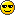Jones

Mensagens : 1
Data de inscrição : 2011-06-26
OuricoDoido, you're my hero, nonless!
Thank you pretty much for your efforts on creating wonderful tutorials like this, having well-explained stuff here and there, let alone exclusive illustrations you have in them as well as deep code analysis.

Since I have been in hacking for quite a while, I was researching information on it for all over the Internet, this led me to the sites like Sonic Retro as well as Sonic Stuff Research Group. Recently, after getting into this mysterious world of hacking classic Sonic games, I've been looking for rather than more and was truly glad to discover your forums.

With no doubts I can consider your work to be more than impressive comparing to the guidelines seen on any other places. You're probably one of the best (if yet not the best) Sonic hacker around the whole hacking scene. Your tutorials open the door into the world of pure high-level hacking and advanced programming, something that wasn't covered by others.

Thank you again for all you do, your work helped me so much!

- Cheers, Jones.Green Snake

Mensagens : 2185
Data de inscrição : 2012-04-07
Localização : I do not even know
Current Project : nopeJoshDP

Mensagens : 1682
Data de inscrição : 2014-10-05
Just an annual year bump!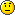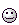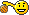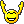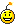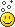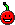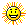:sleep:Just an annual year bump!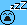Green Snake

Mensagens : 2185
Data de inscrição : 2012-04-07
Localização : I do not even know
Current Project : nope